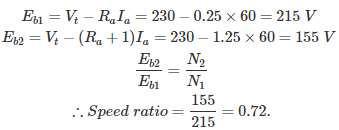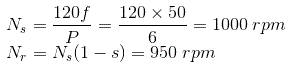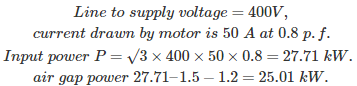# MCQs on Electrical Machines

##### Page 38 of 114. Go to page 1 2 3 4 5 6 7 8 9 10 11 12 13 14 15 16 17 18 19 20 21 22 23 24 25 26 27 28 29 30 31 32 33 34 35 36 37 38 39 40 41 42 43 44 45 46 47 48 49 50 51 52 53 54 55 56 57 58 59 60 61 62 63 64 65 66 67 68 69 70 71 72 73 74 75 76 77 78 79 80 81 82 83 84 85 86 87 88 89 90 91 92 93 94 95 96 97 98 99 100 101 102 103 104 105 106 107 108 109 110 111 112 113 114
01․ When a synchronous motor is running at synchronous speed, the damper winding produces
damping torqe.
eddy current torque.
both 1 and 2.
no torque.

Synchronous motors operate on the basis of magnetic locking tendency. When the rotor is stationary and as the stator poles pass at Ns speed they cannot attract the rotor. Due to damper winding which is also called squirrel cage winding, torque is produced and the rotor rotates near to Ns. After applying DC excitation across the rotor, it forms poles which are rotating at a speed N. At some particular instant rotor north pole and stator south pole gets magnetically locked, rotor steps into synchronism with stator and continues to run at Ns then the damper winding has no value. Because damper winding produces torque only when there is a relative speed between stator and rotor.

02․ If the applied voltage of a DC motor is 230 V, then back emf, for maximum power developed is
115 V.
200 V.
230 V.
460 V.

For maximum power output, the back emf is equal to half of applied voltage. Hence, Eb = Vt/2 = 230/2 ⇒115 V. where Vt is the terminal voltage.

03․ A DC Motor is driving a constant load torque having armature resistance of 0.25 Ω and armature current of 60 A having connected to a voltage of 230 V. Now a resistance of 1 ohm is added into the armature circuit. The ratio of their speed will be
0.62.
1.24.
0.25.
0.72.

For a constant torque load, changing the armature resistance will not change the armature current. So, when the external resistance is added the speed of the motor decreases. The speed of DC motor is proportional to the induced back emf.04․ A three phase 400 V, 6 pole, 50 Hz, squirrel cage induction motor is running at a slip of 5%. The speed of stator magnetic field with respect to rotor magnetic field and speed of rotor w.r.t stator magnetic field are
zero , - 50 rpm.
zero, 950 rpm.
1000 rpm, - 50 rpm.
1000 rpm, 950 rpm.

Stator and rotor magnetic field rotates at synchronous speed i.e. same speed. So difference in speed is zero. Speed of stator magnetic field is Ns and rotor is Nr.So the speed of rotor w.r.t stator magnetic field is Nr – Ns = 950 - 1000 = - 50 rpm. (-) sign indicates that rotor speed is less than the speed of the synchronous magnetic field speed.

05․ A 400 V, 50 Hz, 30 Hp, three phase IM is drawing 50 A current at 0.8 p.f lagging. The stator and rotor copper losses are 1.5 KW and 900 W respectively. The friction and windage losses are 1050 W and core losses are 1200 W. The air gap power of the motor will be
23.06 kW.
24.11 kW.
25 kW.
26.21 kW.

We get air gap power by subtracting the stator copper losses and sator core losses (we can neglect rotor core losses due to low frequency),06․ Synchronous capacitor is
an ordinary static capacitor bank
an over excited synchronous motor driving mechanical load
an over excited synchronous motor without mechanical load
none of the above

When the operating voltages become high and for accurate compensation of reactive power, synchronous condensers are preferred. These are used in all transmission substations and large industries containing large ratings and more number of induction motors operating at low lagging power factor. By installing a synchronous motor and over exciting it,then it acts as a synchronous capacitor and it supplies the required reactive power by the load and reactive power burden on the supply is reduced and automatically improved. Synchronous condenser is connected to a floating system.

07․ For power factor improvement the synchronous motor is to be

Synchronous motor which is over excited operated on no load is known as synchronous condenser. When the excitation across the rotor field winding is increased than the normal value, the motor starts drawing leading current from the supply where it is connected. Drawing leading current means supplying reactive power. When the operating voltages become high and for accurate compensation of reactive power, synchronous condensers are prefered. These are used in all transmission substations and large industries containing large ratings and more number of induction motors operating at low lagging power factor. By installing a synchronous motor and over exciting it,then it acts as a synchronous capacitor and it supplies the required reactive power by the load and reactive power burden on the supply is reduced and automatically improved.

08․ An inverted V-curve of synchronous motor shows the variation of
power factor and dc excitation at constant load
supply voltage and field current at constant excitation
power factor and supply voltage during hunting
all of the above

Inverted V-curve of synchronous motor shows the variation of power factor and dc excitation at constant load. Synchronous motor power factor depends on the excitation value. It acts as a resistor, pure inductor and pure capacitor based on the excitation value. Critical excitation - UPF Under excitation - lagging power factor Over excitation - leading power factor

09․ The basic function of a transformer is to change
the power level
the power factor
the level of the voltage
the frequency

A transformer can be used for either step up or step down the voltages. Its basic application in power system is to transform the voltage from one level to another level without changing its frequency and power.

10․ In a transformer, the frequency of a voltage at the secondary is
greater than the primary.
equal to primary.
less than primary.
any of the above.

The basic function of a transformer is step up or step down voltages without changing its supply frequency. Therefore the frequency of voltage at the secondary is equal to the primary side.

<<<3637383940>>>